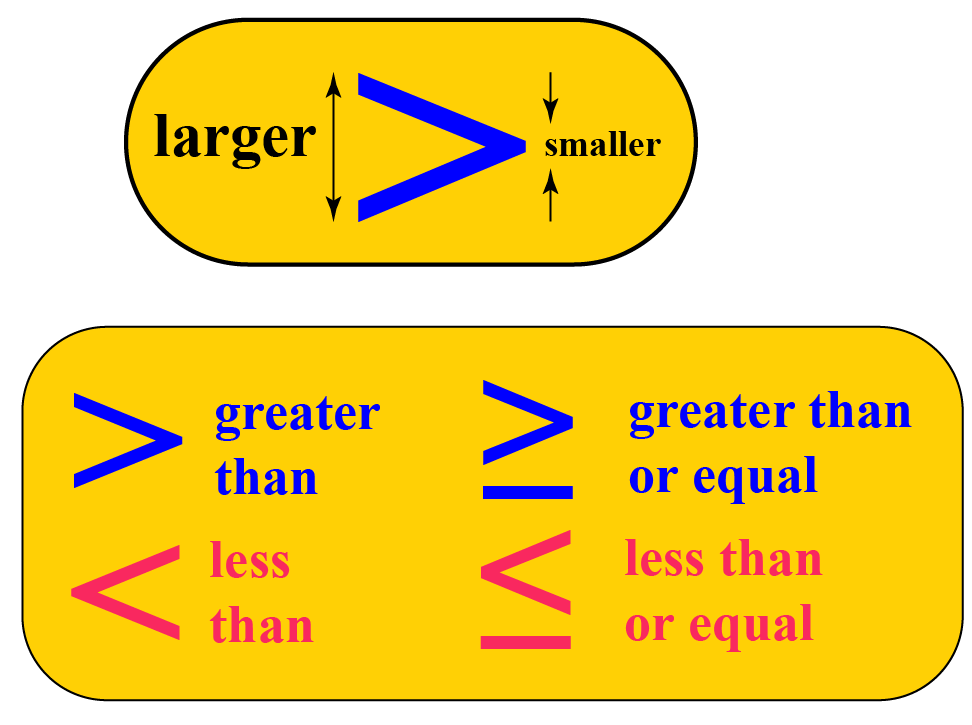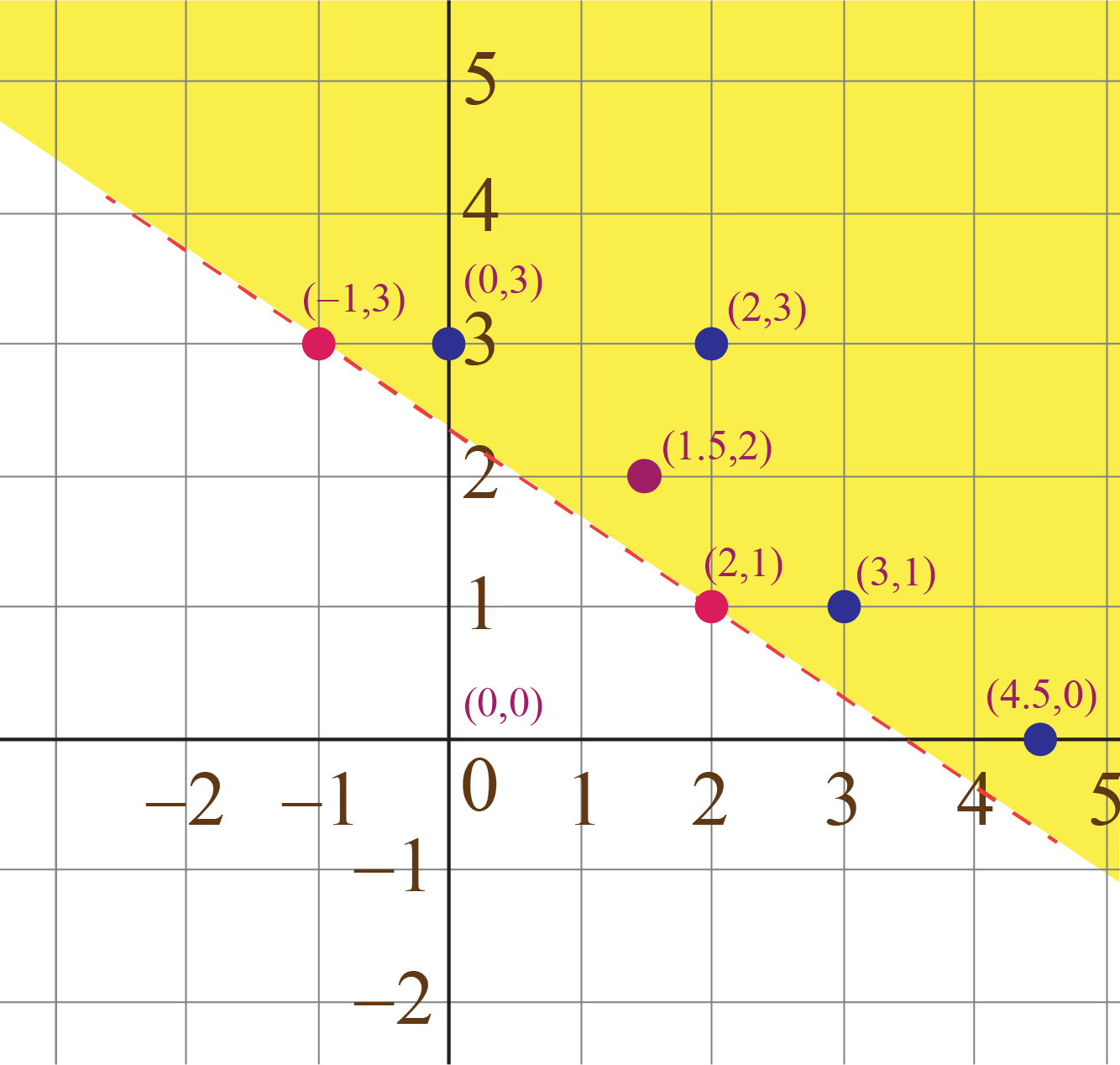# Linear Inequalities in Two Variables

Linear Inequalities in Two Variables

In this mini-lesson, we will learn about infinite sets, ordered pairs, graph linear inequalities in two variables, greater than or equal to, less than or equal to, linear inequalities in two variables and graphing two variable inequalities.

But here's an interesting bit of trivia: Did you know that Thomas Harriot was the person who introduced the concept of inequalities in his book "Artis Analyticae Praxis ad Aequationes Algebraicas Resolvendas" in 1631.## Lesson Plan

 1 What Are Linear Inequalities in Two Variables? 2 Tips and Tricks 3 Important Notes on Linear Inequalities in Two Variables 4 Solved Examples on Linear Inequalities in Two Variables 5 Interactive Questions on Linear Inequalities in Two Variables

## What Are Linear Inequalities in Two Variables?

When one expression is given to be greater than or less than another expression, we have an inequality.

Linear inequalities are defined as expressions where two values are compared using inequality symbols. The symbols representing inequalities are:

Not equal ($$\neq$$)
Less than ($$<$$)
Greater than ($$>$$)
Less than or equal to ($$\leq$$)
Greater than or equal to ($$\geq$$)

Linear inequalities in two variables represent the inequal relationship between two algebraic expressions which includes two distinct variables.

linear inequality in two variables is formed when symbols other than equal to, such as greater than or less than are used to relate two expressions, and two variables are involved.

Here are some examples of linear inequalities in two variables:

$\begin{array}{l}2x< 3y + 2\\7x - 2y > 8\\3x + 4y + 3 \le 2y - 5\\y + x \ge 0\end{array}$

## How Do You Solve Linear Inequalities With Two Variables?

The solution of a linear inequality in two variables, like Ax + By > C, is an ordered pair (x, y) that produces a true statement when the values of x and y are substituted into the inequality.

Solving linear inequalities is the same as solving linear equations; the difference it holds is of inequality symbol.

We solve linear inequalities in the same way as linear equations.

Step 1: Simplify the inequality on both sides, on LHS as well as RHS as per the rules of inequality.
Step 2: Once the value is obtained, we have:

• strict inequalities, in which the two sides of the inequalities cannot be equal to each other.
• non-strict inequalities, in which the two sides of the inequalities can also be equal.

Consider the following inequality:

$2x +3y > 7$

When we talk about finding the solution to this inequality, we are talking about all those pairs of values of x and y for which this inequality is satisfied. This means, for example, that $$x = 4,\;y = 3$$ is one possible solution to this particular inequality. However, $$x = 0,\;y = 0$$ is not, because when you substitute x and y equal to 0 on the LHS of the inequality, it turns out to be less than 7.

We saw that for any linear equation in two variables, there are infinitely many solutions. It might be obvious to you now that for any linear inequality as well, we will have infinitely many solutions. All these solutions will constitute the solution set of the linear inequality.Tips and Tricks
1. PEMDAS and BODMAS play a crucial role in solving inequalities.
2. If the number is negative on either side of a sign (not both), the direction stays the same.

## How Do you Graph Inequalities With Two Variables?

Linear inequalities in two variables have infinite sets or infinitely many ordered pair solutions.

These ordered pairs or the solution sets can be graphed in the appropriate half of a rectangular coordinate plane.

In order to graph inequalities with two variables,

1. Identify the type of inequality (greater than, less than, greater than or equal to, less than or equal to).
2. Graph the boundary line - a dashed (in case of strict inequality) or a solid line (in case of non-strict inequalities).
3. Choose a test point, most probably (0,0) or any other point which is not on the boundary.
4. Shade the region accordingly. If the test point solves the inequality, shade the region that contains it. Otherwise, shade the opposite side of the boundary line.
5. Verify with more number of test points in and out of the region.

Example: Graph the linear equality $2x + 3y > 7$

• Plot the straight line corresponding to the linear equation $$2x + 3y = 7$$.
• Determine any two points (solutions) for this equation:  two possible points on the graph can be taken as $$A\left( { - 1,\;3}\right),\,\,B\left( {2,\;1} \right)$$ and plot them on the graph.
• Determine some specific solutions for the linear inequality $2x + 3y > 7$, which can be as follows \begin{equation}(2,3), (3,1), (4.5,0), (0,3), (1.5,2)\end{equation}
• Plot these five points on the same graph.All the five points (corresponding to the five solutions) lie above the line

• Take any point which lies above the line. Its coordinates, say $$\left( {{x_0},\;{y_0}} \right),$$, will satisfy the inequality: $2{x_0} + 3{y_0} >7$
• This means that the solution set for the inequality consists of all points lying above the line.
• Put x = 0, y = 0, which gives 2(0) + 3(0) > 7, which further gives 0 > 7.This doesn't holds true for the given inequality. So, shade the half plane which doesn't include the point (0,0).
Linear Equations
Linear Equations
grade 9 | Questions Set 2
Linear Equations
Linear Equations
grade 9 | Questions Set 1
More Important Topics
Numbers
Algebra
Geometry
Measurement
Money
Data
Trigonometry
Calculus
More Important Topics
Numbers
Algebra
Geometry
Measurement
Money
Data
Trigonometry
Calculus